# How to use IF function in Excel: examples for text, numbers, dates, blanks

In this article, you will learn how to build an Excel IF statement for different types of values as well as how to create multiple IF statements.

IF is one of the most popular and useful functions in Excel. Generally, you use an IF statement to test a condition and to return one value if the condition is met, and another value if the condition is not met.

In this tutorial, we are going to learn the syntax and common usages of the Excel IF function, and then take a closer look at formula examples that will hopefully prove helpful to both beginners and experienced users.

## IF function in Excel

IF is one of logical functions that evaluates a certain condition and returns one value if the condition is TRUE, and another value if the condition is FALSE.

The syntax of the IF function is as follows:

IF(logical_test, [value_if_true], [value_if_false])

As you see, IF takes a total of 3 arguments, but only the first one is obligatory, the other two are optional.

Logical_test (required) - the condition to test. Can be evaluated as either TRUE or FALSE.

Value_if_true (optional) - the value to return when the logical test evaluates to TRUE, i.e. the condition is met. If omitted, the value_if_false argument must be defined.

Value_if_false (optional) - the value to return when the logical test evaluates to FALSE, i.e. the condition is not met. If omitted, the value_if_true argument must be set.

## Basic IF formula in Excel

To create a simple If then statement in Excel, this is what you need to do:

• For logical_test, write an expression that returns either TRUE or FALSE. For this, you'd normally use one of the logical operators.
• For value_if_true, specify what to return when the logical test evaluates to TRUE.
• For value_if_false, specify what to return when the logical test evaluates to FALSE. Though this argument is optional, we recommend always configuring it to avoid unexpected results. For the detailed explanation, please see Excel IF: things to know.

As an example, let's write a very simple IF formula that checks a value in cell A2 and returns "Good" if the value is greater than 80, "Bad" otherwise:

`=IF(B2>80, "Good", "Bad")`

This formula goes to C2, and then is copied down through C7: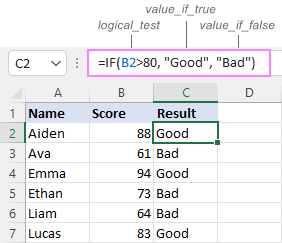In case you wish to return a value only when the condition is met (or not met), otherwise - nothing, then use an empty string ("") for the "undefined" argument. For example:

`=IF(B2>80, "Good", "")`

This formula will return "Good" if the value in A2 is greater than 80, a blank cell otherwise: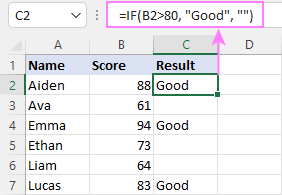## Excel If then formula: things to know

Though the last two parameters of the IF function are optional, your formula may produce unexpected results if you don't know the underlying logic.

### If value_if_true is omitted

If the 2nd argument of your Excel IF formula is omitted (i.e. there are two consecutive commas after the logical test), you'll get zero (0) when the condition is met, which makes no sense in most cases. Here is an example of such a formula:

`=IF(B2>80, , "Bad")`

To return a blank cell instead, supply an empty string ("") for the second parameter, like this:

`=IF(B2>80, "", "Bad")`

The screenshot below demonstrates the difference: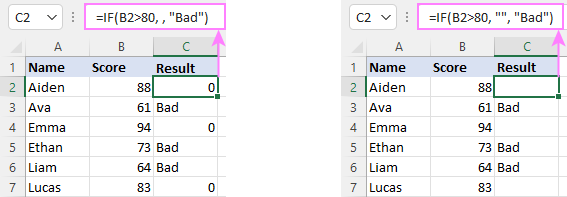### If value_if_false is omitted

Omitting the 3rd parameter of IF will produce the following results when the logical test evaluates to FALSE.

If there is just a closing bracket after value_if_true, the IF function will return the logical value FALSE. Quite unexpected, isn't it? Here is an example of such a formula:

`=IF(B2>80, "Good")`

Typing a comma after the value_if_true argument will force Excel to return 0, which doesn't make much sense either:

`=IF(B2>80, "Good",)`

The most reasonable approach is using a zero-length string ("") to get a blank cell when the condition is not met:

`=IF(B2>80, "Good", "")`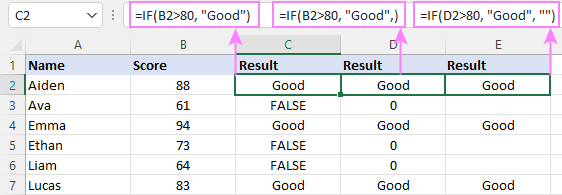Tip. To return a logical value when the specified condition is met or not met, supply TRUE for value_if_true and FALSE for value_if_false. For the results to be Boolean values that other Excel functions can recognize, don't enclose TRUE and FALSE in double quotes as this will turn them into normal text values.

## Using IF function in Excel - formula examples

Now that you are familiar with the IF function's syntax, let's look at some formula examples and learn how to use If then statements in real-life scenarios.

### Excel IF function with numbers

To build an IF statement for numbers, use logical operators such as:

• Equal to (=)
• Not equal to (<>)
• Greater than (>)
• Greater than or equal to (>=)
• Less than (<)
• Less than or equal to (<=)

Above, you have already seen an example of such a formula that checks if a number is greater than a given number.

And here's a formula that checks if a cell contains a negative number:

`=IF(B2<0, "Invalid", "")`

For negative numbers (which are less than 0), the formula returns "Invalid"; for zeros and positive numbers - a blank cell.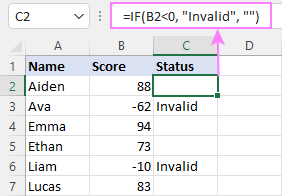### Excel IF function with text

Commonly, you write an IF statement for text values using either "equal to" or "not equal to" operator.

For example, the following formula checks the Delivery Status in B2 to determine whether an action is required or not:

`=IF(B2="delivered", "No", "Yes")`

Translated into plain English, the formula says: return "No" if B2 is equal to "delivered", "Yes" otherwise.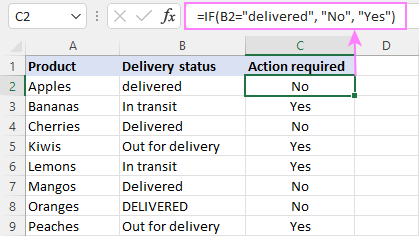Another way to achieve the same result is to use the "not equal to" operator and swap the value_if_true and value_if_false values:

`=IF(C2<>"delivered", "Yes", "No")`

Notes:

• When using text values for IF's parameters, remember to always enclose them in double quotes.
• Like most other Excel functions, IF is case-insensitive by default. In the above example, it does not differentiate between "delivered", "Delivered", and "DELIVERED".

### Case-sensitive IF statement for text values

To treat uppercase and lowercase letters as different characters, use IF in combination with the case-sensitive EXACT function.

For example, to return "No" only when B2 contains "DELIVERED" (the uppercase), you'd use this formula:

`=IF(EXACT(B2,"DELIVERED"), "No", "Yes")`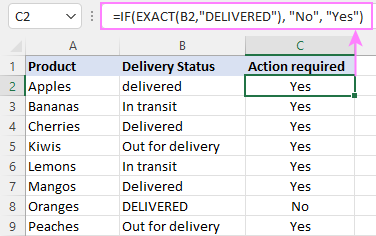### If cell contains partial text

In situation when you want to base the condition on partial match rather than exact match, an immediate solution that comes to mind is using wildcards in the logical test. However, this simple and obvious approach won't work. Many functions accept wildcards, but regrettably IF is not one of them.

A working solution is to use IF in combination with ISNUMBER and SEARCH (case-insensitive) or FIND (case-sensitive).

For example, in case "No" action is required both for "Delivered" and "Out for delivery" items, the following formula will work a treat:

`=IF(ISNUMBER(SEARCH("deliv", B2)), "No", "Yes")`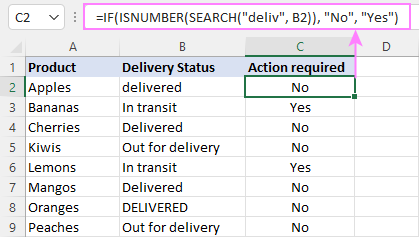### Excel IF statement with dates

At first sight, it may seem that IF formulas for dates are akin to IF statements for numeric and text values. Regrettably, it is not so. Unlike many other functions, IF does recognize dates in logical tests and interprets them as mere text strings. In other words, you cannot supply a date in the form of "1/1/2020" or ">1/1/2020". To make the IF function recognize a date, you need to wrap it in the DATEVALUE function.

For example, here's how you can check if a given date is greater than another date:

`=IF(B2>DATEVALUE("7/18/2022"), "Coming soon", "Completed")`

This formula evaluates the dates in column B and returns "Coming soon" if a game is scheduled for 18-Jul-2022 or later, "Completed" for a prior date.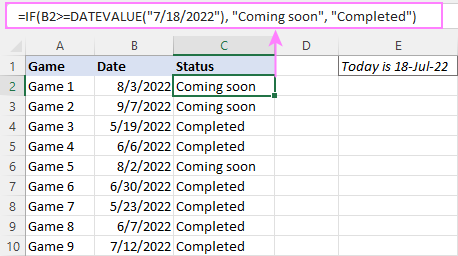Of course, there is nothing that would prevent you from entering the target date in a predefined cell (say E2) and referring to that cell. Just remember to lock the cell address with the \$ sign to make it an absolute reference. For instance:

`=IF(B2>\$E\$2, "Coming soon", "Completed")`

To compare a date with the current date, use the TODAY() function. For example:

`=IF(B2>TODAY(), "Coming soon", "Completed")`

### Excel IF statement for blanks and non-blanks

If you are looking to somehow mark your data based on a certain cell(s) being empty or not empty, you can either:

• Use the IF function together with ISBLANK, or
• Use the logical expressions ="" (equal to blank) or <>"" (not equal to blank).

The table below explains the difference between these two approaches with formula examples.

 Logical test Description Formula Example Blank cells ="" Evaluates to TRUE if a cell is visually empty, even if it contains a zero-length string. Otherwise, evaluates to FALSE. =IF(A1="", 0, 1) Returns 0 if A1 is visually blank. Otherwise returns 1. If A1 contains an empty string (""), the formula returns 0. ISBLANK() Evaluates to TRUE is a cell contains absolutely nothing - no formula, no spaces, no empty strings. Otherwise, evaluates to FALSE. =IF(ISBLANK(A1), 0, 1) Returns 0 if A1 is absolutely empty, 1 otherwise. If A1 contains an empty string (""), the formula returns 1. Non-blank cells <>"" Evaluates to TRUE if a cell contains some data. Otherwise, evaluates to FALSE. Cells with zero-length strings are considered blank. =IF(A1<>"", 1, 0) Returns 1 if A1 is non-blank; 0 otherwise. If A1 contains an empty string, the formula returns 0. ISBLANK()=FALSE Evaluates to TRUE if a cell is not empty. Otherwise, evaluates to FALSE. Cells with zero-length strings are considered non-blank. =IF(ISBLANK(A1)=FALSE, 0, 1) Works the same as the above formula, but returns 1 if A1 contains an empty string.

And now, let's see blank and non-blank IF statements in action. Suppose you have a date in column B only if a game has already been played. To label the completed games, use one of these formulas:

`=IF(B2="", "", "Completed")`

`=IF(ISBLANK(B2), "", "Completed")`

`=IF(\$B2<>"", "Completed", "")`

`=IF(ISBLANK(\$B2)=FALSE, "Completed", "")`

In case the tested cells have no zero-length strings, all the formulas will return exactly the same results: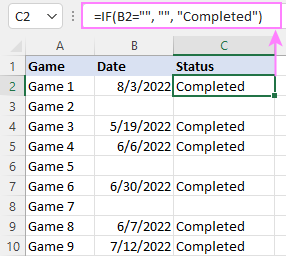### Check if two cells are the same

To create a formula that checks if two cells match, compare the cells by using the equals sign (=) in the logical test of IF. For example:

`=IF(B2=C2, "Same score", "")`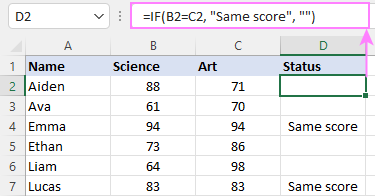To check if the two cells contain same text including the letter case, make your IF formula case-sensitive with the help of the EXACT function.

For instance, to compare the passwords in A2 and B2, and returns "Match" if the two strings are exactly the same, "Do not match" otherwise, the formula is:

`=IF(EXACT(A2, B2), "Match", "Don't match")`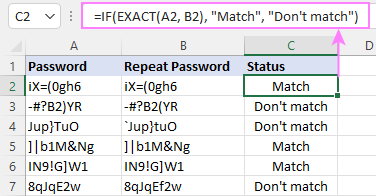### IF then formula to run another formula

In all of the previous examples, an Excel IF statement returned values. But it can also perform a certain calculation or execute another formula when a specific condition is met or not met. For this, embed another function or arithmetic expression in the value_if_true and/or value_if_false arguments.

For example, if B2 is greater than 80, we'll have it multiplied by 7%, otherwise by 3%:

`=IF(B2>80, B2*7%, B2*3%)`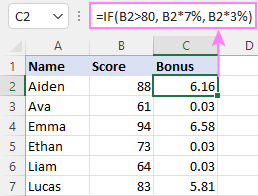## Multiple IF statements in Excel

In essence, there are two ways to write multiple IF statements in Excel:

• Nesting several IF functions one into another
• Using the AND or OR function in the logical test

### Nested IF statement

Nested IF functions let you place multiple IF statements in the same cell, i.e. test multiple conditions within one formula and return different values depending on the results of those tests.

Assume your goal is to assign different bonuses based on the score:

• Over 90 - 10%
• 90 to 81 - 7%
• 80 to 70 - 5%
• Less than 70 - 3%

To accomplish the task, you write 3 separate IF functions and nest them one into another like this:

`=IF(B2>90, 10%, IF(B2>=81, 7%, IF(B2>=70, 5%, 3%)))`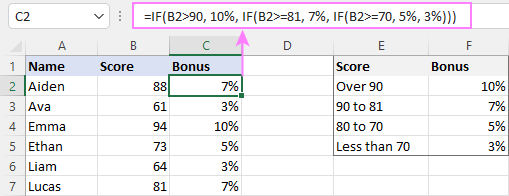For more formula examples, please see:

### Excel IF statement with multiple conditions

To evaluate several conditions with the AND or OR logic, embed the corresponding function in the logical test:

For example, to return "Pass" if both scores in B2 and C2 are higher than 80, the formula is:

`=IF(AND(B2>80, C2>80), "Pass", "Fail")`

To get "Pass" if either score is higher than 80, the formula is:

`=IF(OR(B2>80, C2>80), "Pass", "Fail")`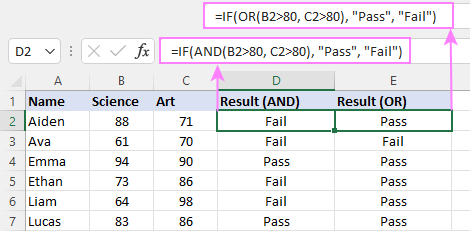## If error in Excel

Starting from Excel 2007, we have a special function, named IFERROR, to check formulas for errors. In Excel 2013 and higher, there is also the IFNA function to handle #N/A errors.

And still, there may be some circumstances when using the IF function together with ISERROR or ISNA is a better solution. Basically, IF ISERROR is the formula to use when you want to return something if error and something else if no error. The IFERROR function is unable to do that as it always returns the result of the main formula if it isn't an error.

For example, to compare each score in column B against the top 3 scores in E2:E4, and return "Yes" if a match is found, "No" otherwise, you enter this formula in C2, and then copy it down through C7:

`=IF(ISERROR(MATCH(B2, \$E\$2:\$E\$4, 0)), "No", "Yes" )`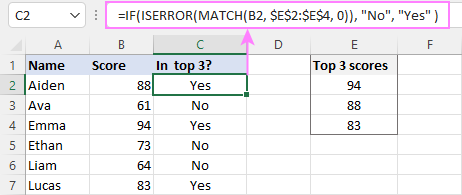Hopefully, our examples have helped you get a grasp of the Excel IF basics. I thank you for reading and hope to see you on our blog next week!

## Practice workbook

Excel IF statement - formula examples (.xlsx file)

## You may also be interested in

1. I'm using a formula "=TEXTJOIN("",TRUE,IFERROR((MID(C39,ROW(INDIRECT("1:"&LEN(C39))),1)*1),""))" to copy numeric contents of a cell to another cell, now I would like to modify it to the same but only if it is 9 digits or more if it is less than 9 digits to copy the complete cell

• Hi! If I understand correctly, use the LEN function to find the length of the text string that TEXTJOIN returns.

=IF(LEN(TEXTJOIN("",TRUE,IFERROR((MID(C39,ROW(INDIRECT("1:"&LEN(C39))),1)*1),"")))>9, C39, TEXTJOIN("",TRUE,IFERROR((MID(C39,ROW(INDIRECT("1:"&LEN(C39))),1)*1),"")))

2. Please help to create an If statement formula identifying the Peak (P) and Off peak (OP) in the time of a day with the following conditions:
Peak (P) - from 0801H - 2100H Mon-Sat and 1801H-2100H during Sundays and holidays
Off Peak (OP) - from 2101H-0800H Mon-Sat and 2101H-1800H during Sundays and holidays
Tried so many formulas but returns a #value. 26/12/2022 12:05:00 am is the date-time format reference that Iam using.
Thank you.

• Hi! To get the day of the week, use the WEEKDAY function. Since time in Excel is the fractional part of the date, get this fractional part by subtracting the integer part from the date using the INT function.

=IF(OR(AND(WEEKDAY(D1,2)<7, (D1-INT(D1))>TIME(8,0,0), (D1-INT(D1))<TIME(21,0,0)), AND(WEEKDAY(D1,2)=7, (D1-INT(D1))>TIME(18,0,0), (D1-INT(D1))<TIME(21,0,0))),"P","OP")

3. helloo Dear Alexander

I need a function a liittle unusual. just wanted to ask you to help me.
=IF(Condition = TRue, condition1, get data from user)

in other word If condition is True do an affair an calculation, but if is not true, get as data entry.

would you please to help me if there is a way to do this?

Thanks alot.

• Hi! What does the “get data from user” phrase mean? If you want to get data using an external reference, specify it in the IF formula. If you want to get data for multiple cells or a table, use VBA.

4. Hello!in sheet1 from column A - K are all occupied,in column G there are mixture up trucks registrations numbers of 3 different countries,i need to maintain that column but link every country registration number to go to different sheet depending on first letter of the registration

5. Hi,
Can i use if formula "Monthly data same date if there is same name is enter double then it should be highlighted "i dont want to filter and check each date if the name is double entry or not

• Unfortunately, I can't guess what formula you are talking about or what you want to do.

• My concern is I have a data with many names and different date's

A with name and B with date

Cell "A " if the name is entered 2 times on the same date .
example: name John - 1st oct 2022 is OK ,should not be there again in the same date .

i need a formula to confirm there is no double entry on the same date

• Hi! If I understand your task correctly, the following tutorial should help: Highlight duplicate rows in Excel.
You can use a formula to indicate a duplicate record:

=IF(COUNTIFS(\$A\$2:\$A\$100,\$A2,\$B\$2:\$B\$1100,\$B2)>1,"Duplicate","")

6. Can I return a number and a text value? I am trying to get the value in the cell with the IF statement to display the numerical difference between two cells and also say Under or Over. For context, I want it to subtract an estimated budget with an actual budget and then return the value saying how much the difference is and if it is over or under budget in the same cell. Is this possible?

7. Hi
How to write this formula:
If cell B4 is >1 or =15 then B5=1
If cell B4 is <15 but < 30 then B5=2
If cell B4 is 305 but < 45 then B5=3

Ihave been trying to use IF(AND/ OR but I am not getting it write.

8. Mohonbantuan untukrumusnya
jika A1 saya isi P maka A2 isinya 100.000, jika A1 disi T maka A2 isinya 200.000, jika A1 di isi K maka A2 300.000

mohon bantuannya, trus cara aturnya gimana.
terimasaik,

• I want the numbers to showi the first column automatically, as i enter the names or items in the next column. How can i do that? I remember something like: =if(b2=???, row-1, ""), but cannot remember the exact formula.

• Hi! I don't know what numbers you want to display. You can get the row number by using the ROW function. Check for an empty cell using the ISBLANK function or the <>"" expression. For example:

=IF(NOT(ISBLANK(B2)),ROW(B2),"")
=IF(B2<>"",ROW(B2),"")

9. Hi!
I have tax rate ranging from 5% - 20% and each are give the rate

5,000 is nil tax
5,001 - 10,000 is 5%
10,001-15,000 is 10%
15,001 - 20,000 is 15%
20,001- above is 20%

How can this be incorporate in the formula?

10. I have 2 columns. One of the column is labeled "Earnings Overpriced". In that column I have successfully calculated either "Overpriced" or nothing appears in the cells. In the second column labeled "Cash Flow Overpriced". In that column I have successfully calculated either "Overpriced" or nothing appears in the cells. In a third column I want excel to look at both these column cells and when "Overpriced" appears in both columns I want excel to calculated in the third column "Earnings and Cash Flow Overpriced". When the "Overpriced" appears in only the Earnings column and the Cash Flow column has nothing in the cell, I want excel to calculate "Earnings Overpriced". When the "Overprice" appears only in the Cash Flow column but the Earnings column has nothing in it, I want excel to calculate "Cash Flow Overpriced". When both columns have nothing in them, I would like excel to place a "Buy" in the cell. This seems simple to me but I can't get my formula to work.

11. Hello,

- Column A contains % change values that are positive and negative. Column A has rows that contain no values.

-I'd like to display the % change values that are positive in Column B and nothing if a Column A row contains no value.
-I'd like to display the % change values that are negative in Column C and nothing if a Column A row contains no value.

Thank you very much!

• Hi! Please re-check the article above since it covers your task. If I understand correctly, thy this formula

IF(A1>0,A1,"")

12. Hi there!
Loved using this for my basic data entry but now i have a super complicated entry! (in my mind at least)

I have multiple entries containing different "finishes" eg Black 16mm in A B C D E
I need a cell to basically run a bunch of formulas and add text where the values are matching
for example i have a black 16mm board but i have 5 different finishes for this board - we'll call them A, B, C, D, E
Can I get a single cell formula to basically look at all these values (A-E) and spit out the value i need like "Black 16mm C"

I currently have a formula saying if "A" then TEXT, if not ATTENTION but having to do this for many seperate values is time consuming!

• Hi! You can use the drop-down list to view the list of values and select the desired value from it. I hope it’ll be helpful.

13. I compare values in two columns of a row and using ÍF" condition I confirms whether they are same.
But , even ifthe values are same, the Ïf"condition formula returns "False" in certain cases( not in all cases).Why it is so?

14. I need to write a formula that states if a values multiplied by another value plus a value divided by 2 is less than a value in a cell it will equal that number in the cell plus 1

• I hope you have studied the recommendations in the tutorial above. It contains answers to your question.

15. Hi,
I have a value in cell b1("Date") and b2("Transferred"). If B2=Transferred, then b1 and b2 must return a blank values
If b1 and b2 have no values, then cells remains blank
if b1("date") and B2 says Dismissed or Resigned, then both cells stay as is.
Thank you

• I don't understand how B2 can simultaneously have the value "Transferred" and return an blank value. Explain.

16. A2, B2 & C2 having NA then result come delete or A2, B2 & C2 HAVING OTHER VALUE THEN result come no action

• I am not sure I fully understand what you mean.

17. Help. Can you help me with a formula for this scenario:

If a number is between 40,000-83,999 the multiply that number by 15% or 8,399, whichever is less, if a number is between 84,000-124000 then multiply that number by 10% or 9,999, which is less...

Thank you so much if you can help me with this.

• Hi! Use the nested IF function. Find the minimum value with the MIN function.

=IF(AND(A1>40000,A1<83999),MIN(A1*0.15,8399),IF(AND(A1>84000,A1<124000),MIN(A1*0.1,9999),""))

18. This is a fantastic resource. I am diving into making better spreadsheets at work, and already enjoying conditional logic built into Excel even though we are using MS Excel 2010.

I have a spreadsheet that I have made for job numbers. I have a "due date" in Column Cell D3 which is using icon sets to place a red circle icon and second condition for the same cell to have format of the cell shaded red. This works well for a visual of when the date has reached and is now expired, the visual is perfect.

However, I have another column with "Completed date" which is a manual entry in column Cell K3. I would like that when we add a date in K3, this is a trigger to change the icon set to use a green tick icon, and then change the shading of the background to green.

I cannot work out the conditional logic for this scenario.

Many thanks

• Hi! Create a conditional formatting formula, where you use the logical AND function to combine the conditions for cells D3 and K3.
The formula might look like this:

=AND([conditions for Cell D3],(ISNONTEXT(K3),K3>0)